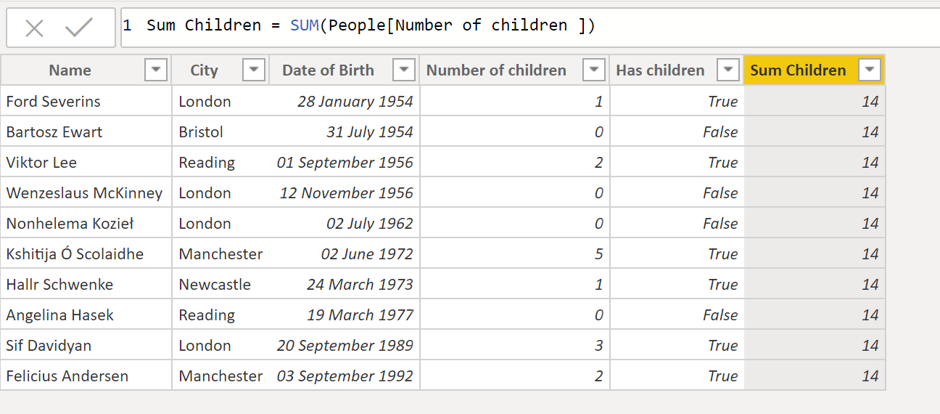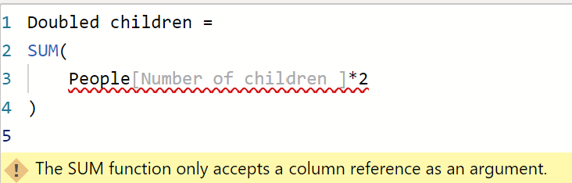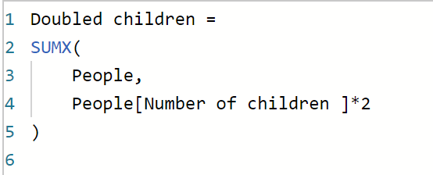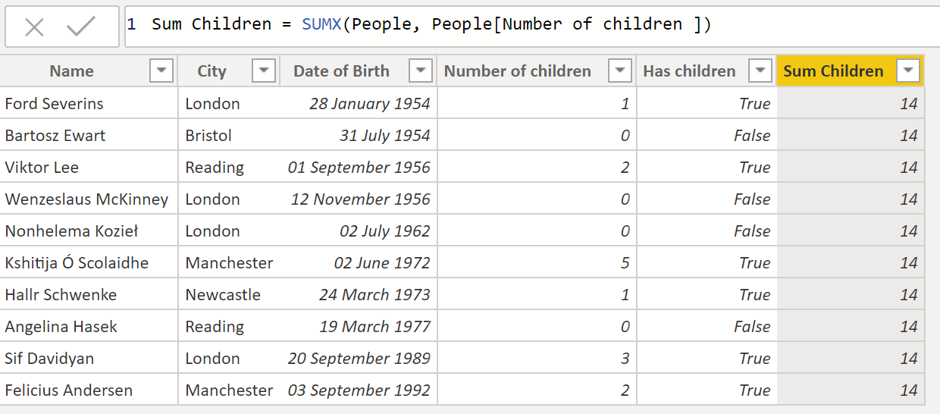So far I have covered filter contexts, row contexts, and the differences between calculated columns and measures. I next want to focus on aggregation functions, which are the building blocks for a huge amount of more complex processing.

There are many aggregation functions, but the most commonly used are:

• `SUM`
• `AVERAGE`
• `MIN`
• `MAX`

These operators allow you to aggregate values for an individual column and they will take into account the filter context under which they operate, as the dataset they work over will be the one defined by this context.They do, however, ignore the row context. To show you what I mean, lets create a calculated column in the people table:We can see that the same value has been added to each row of the table. This is because the aggregate operators are ignoring the row context under which they are operating (which relates to the current row of iteration), and there is no filter context applied in the raw data view so all of the data is summed.The aggregation operators are used extensively with measures, to get e.g. the total children, the average number of children, etc.

## X-Aggregators

The previous operators can only work on a simple column. You can't, for example, do:For this you need to iterate over each row, do the evaluation, and then `SUM` the results.You could do this by using a calculated column, but that would cause the report to use more memory every time you wanted to do a computed aggregation… Luckily there is another option:The first argument here is the table over which to operate and the second is the expression which you want to `SUM`. This will still take into account the filter context under which the aggregator is applied as this context will define the dataset over which the aggregator is operating and therefore the rows over which to iterate. This produces a doubled `SUM` of the number of children:Notice here that I have used the word iterate. These operators use row contexts in the same way as a calculated column to iterate over each individual row, execute the formula for that row, and then finally `SUM` the results.

It is important to highlight that these X-aggregators still don't take into account the row context when doing the aggregation:I think it's best to think of the X-aggregators in two steps (which both happen under whatever filter context is currently applied):

1. Create a temporary calculated column (which uses the row context to evaluate the expression for each row)
2. Use the `SUM` operator on the temporary calculated column

Using these X-aggregators, we can build up quite complex processing, especially when combined with table functions, which we will see in the next blog!

### Carmel Eve

#### Software Engineer I